C - Linked List                                        Home : www.sharetechnote.com Linked List   Linked List is a kind of data structure that is designed to store multiple elements in a single variable. In terms of the purpose, you may think it is similar to an Array. But there is difference between an Array and LinkedList in terms of implementation. The biggest difference can be illustrated as below. In an array, the program allocate a consecutive memory blocks to store the items, but in linked list the location of each items may be scattered around here and there. Another difference is .. when you define an array, you should know the size of memory (the number of the elements) when you declair the variable, but in linked list you don't have to know this when you create the list. You would add or delete memory space as it is necessary.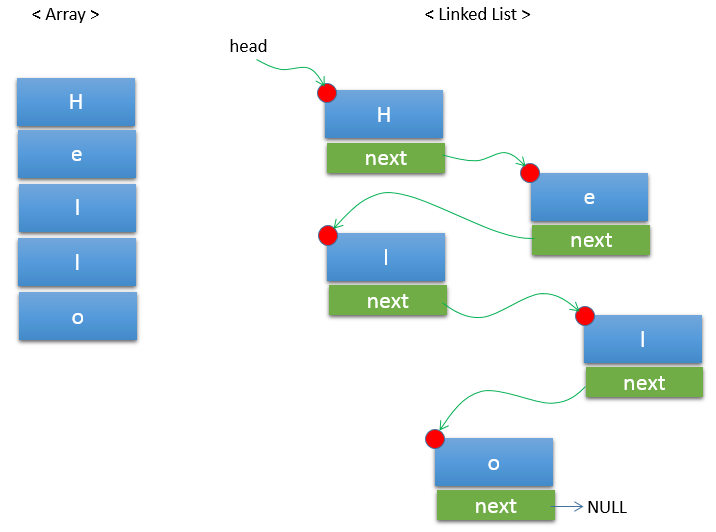Now you may ask when do I have to use Array and when do I have to use Linked List ? Actually there is no clear/solid answer for this, but I may recommend i) to use an array if we are sure that you have enough memory to allocate consecutively to store all the information in the array ii) to use LinkedArray if you need to do a lot of insertion and deletion operation of the elements. (For example, let's suppose that you want to insert a character in the middle of 'Hello' in the array shown above. It is not impossible, but you have to do a lot of tricks to do this. Just try this. However, this kind of operation can be easily done in LinkedList. You will see this example later). iii) there are some cases that it is almost impossible to implement with an array. For example, it would be almost impossible to create a structure like a tree or graph with an array. In this case, LinkedList would be the best option. It sounds like LinkedList is pretty useful method. However, the problem is that it is not easy to understand the concept and even harder to write a code for this. Probably this would be one of the most challenging one for beginers. At least, it has been very challenging to me.. it took me several years for me to FEEL comfortable on this .. but still confusing if I need to implement this without any cheatsheet :). So, don't get disappointed if you don't understand this right away .. just try with examples as many as possible. Don't just try to copy the source code made by other.. try to analyze those example line by line and visualize/verbalize in your own way.   Now let's take a loot at a couple of simple examples of LinkedList. Before you go through this example, you should be familiar with the concept of Ponters. So if you are not so familiar with Pointers, it would be a good idea to study about Pointers first.   Example 1 > ----------------------------------------------------------------------------------------------   This can be one of the simplest example for Linked List. I don't think there would be anybody who will implement a LinkedList in such an inefficient way like this. But I wrote in this kind of inefficient way just to help you to understand the process more clearly and in step by step. You will do the exactly same job but in a little bit more efficient way in Example 2.   Following is the source code for the first example. First take a look at the code and comments and check if it make sense to you. Try to visualize each and every step on your own and then go through the explanation at the end.   #include #include   //(1) Define a structure of the node that is used to construct a list struct charList {     char letter;     struct charList *next; };   //(2) Define variables that are used in the process of building up a list struct charList *head = NULL; struct charList *curr = NULL; struct charList *node = NULL;     int main(int argc, char* argv[]) {       //(3) reserve a memory block with the size of charList and assign the address to the variable (pointer) 'node'     node = (struct charList*)malloc(sizeof(struct charList));       //(4) assign a character 'H' to the letter field of the node and set 'NULL' to the next field of the node'     node->letter = 'H';     node->next = NULL;       //(5) Make all the variable head, curr, node point to the same location     head = curr = node;          //(6) reserve a memory block with the size of charList and assign the address to the variable (pointer) 'node'     node = (struct charList*)malloc(sizeof(struct charList));       //(7) assign a character 'e' to the letter field of the 'node' and set 'NULL' to the next field of the 'node'     node->letter = 'e';     node->next = NULL;       //(8) Make 'next' pointer of curr node point to 'node''     curr->next = node;       //(9) Make  the 'curr' point to 'node''     curr=node;       //(10) reserve a memory block with the size of charList and assign the address to the variable (pointer) 'node'     node = (struct charList*)malloc(sizeof(struct charList));       //(11) assign a character 'l' to the letter field of the 'node' and set 'NULL' to the next field of the 'node'     node->letter = 'l';     node->next = NULL;          //(12) Make 'next' pointer of curr node point to 'node''     curr->next = node;       //(13) Make  the 'curr' point to 'node'     curr=node;       //(14) reserve a memory block with the size of charList and assign the address to the variable (pointer) 'node'     node = (struct charList*)malloc(sizeof(struct charList));       //(15) assign a character 'l' to the letter field of the 'node' and set 'NULL' to the next field of the 'node'     node->letter = 'l';     node->next = NULL;          //(16) Make 'next' pointer of curr node point to 'node''     curr->next = node;       //(17) Make  the 'curr' point to 'node'     curr=node;       //(18) reserve a memory block with the size of charList and assign the address to the variable (pointer) 'node'     node = (struct charList*)malloc(sizeof(struct charList));       //(19) assign a character 'o' to the letter field of the 'node' and set 'NULL' to the next field of the 'node'     node->letter = 'o';     node->next = NULL;          //(20) Make 'next' pointer of curr node point to 'node''     curr->next = node;       //(21) Make  the 'curr' point to 'node'     curr=node;       //(22) Print all the list       node = head;       printf("\n -------Printing the list ------- \n");       while(node != NULL)     {         printf("%c",node->letter);         node = node->next;     };          return 0; }   Result > -------Printing the list ------- Hello   Now let's go through each line of the source code and think of what would happen in the process and the computer memory. Even though it is described in picture, it might not be clear to you if you are not familiar with Pointer. And even if you successfully understand this process while you are reading this page, it may confuse you again when you try to code the program on your own. Again, don't get disappointed with this.. it is normal.. just try again and again ... and again.   This is the first line to look into. I assume that you know what malloc() function does. Here it allocates a memory block with the size of 'struct charList' and assign the pointer(address, expressed in red point below)) to the variable 'node'. With this, the pointer (variable) node points to the address of the new memory block as shown below.   node = (struct charList*)malloc(sizeof(struct charList));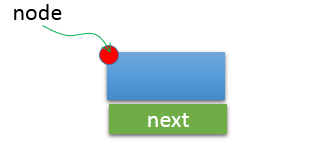Now we run following codes. These are just an assignment process. By the first line, the letter 'H' is stored in the struct 'node' and by the second line the element 'next' (declaired as a pointer) is assigned with 'NULL'. It means 'next' does not points to anywhere.   node->letter = 'H'; node->next = NULL;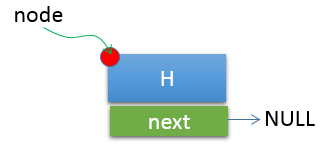Now we see following line. With this, all the three pointer variables (head, curr, node) points to the same location of the memory as shown below.   head = curr = node;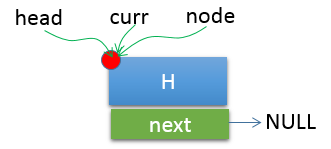Now we have another malloc() as shown below. With this, a new memory block gets reserved and assigned to the variable 'node'. Now head, curr are still pointing to the previous block and node is pointing to a new block.   node = (struct charList*)malloc(sizeof(struct charList));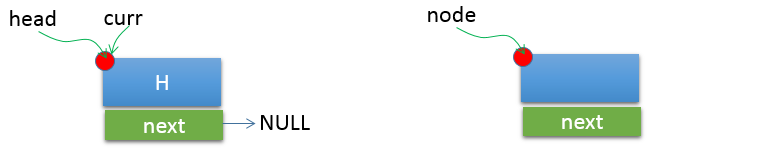Now we have another assignment routine as shown below. With this, the memory block on the right are assigned with values as shown below.   node->letter = �e'; node->next = NULL;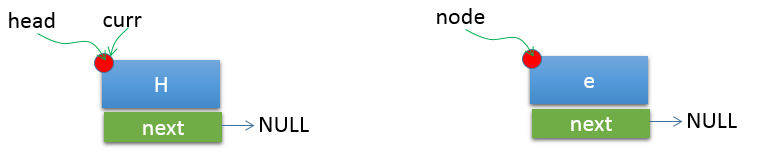At this point, you have two memory block and all of them are assigned with its own value, but the two memory blocks are separate (meaning 'NOT LINKED').   Now we get a very important step. This means let the next element of curr block points to the memory block 'node'. By this step, the two memory blocks get connected (linked) as shown below.   curr->next = node;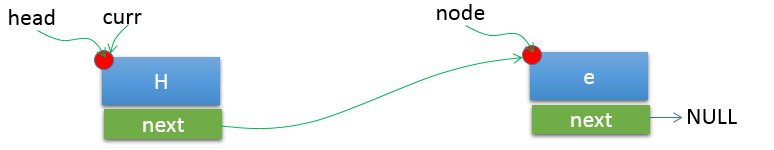Now this is the last step of the first link. At the last step of every addition of new elements comes as follows. With this, the 'curr' variable gets to point to the last elements in the linked list that has been created as of now. Also, note that the variable 'head' is still pointing to the first elements in the linked list.   curr = node;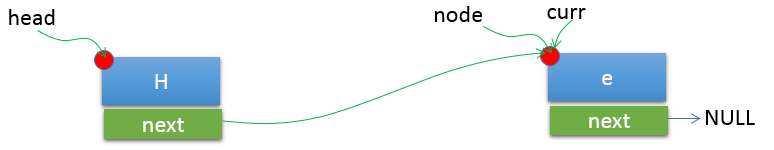From now, the same process goes over and over.   Here, we have another malloc() as shown below. With this, a new memory block gets reserved and assigned to the variable 'node'. Now head, curr are still pointing to the previous block and the 'node' is pointing to a new block.   node = (struct charList*)malloc(sizeof(struct charList));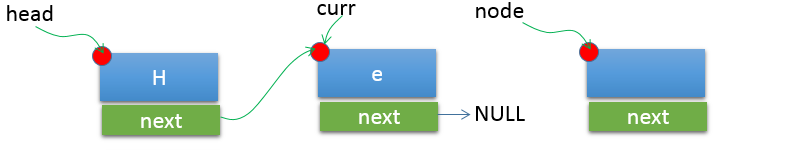Then, we have another assignment routine as shown below. With this, the memory block on the right are assigned with values as shown below.   node->letter = �l'; node->next = NULL;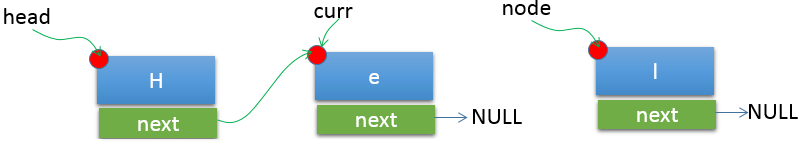At this point, you have three memory blocks and all of them are assigned with its own value. Two of the blocks are linked and the last one is not linked yet.   Now we get another linking step. This means let the next element of curr block points to the memory block 'node'. By this step, the third memory blocks get connected (linked) to the previous link  and now all of the three blocks got connected as shown below.   curr->next = node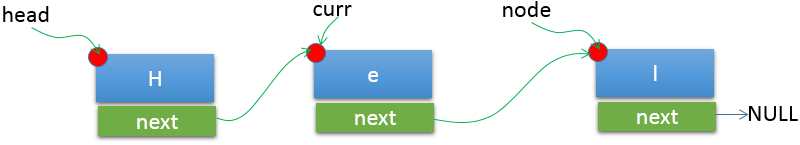Now we reached the last step of the second link. With this, the 'curr' variable gets to point to the last elements in the linked list that has been created as of now. Also, note that the variable 'head' is still pointing to the first elements in the linked list.   curr = node;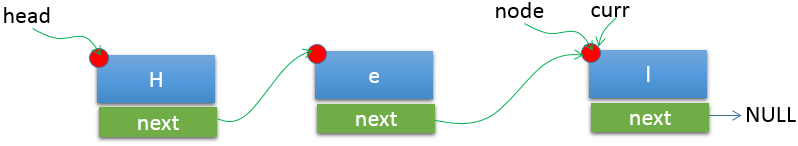I hope you get the logic of this process and can complete the remaining steps on your own. If you successfully followed up all the step, you should get the status as shown below after step (21).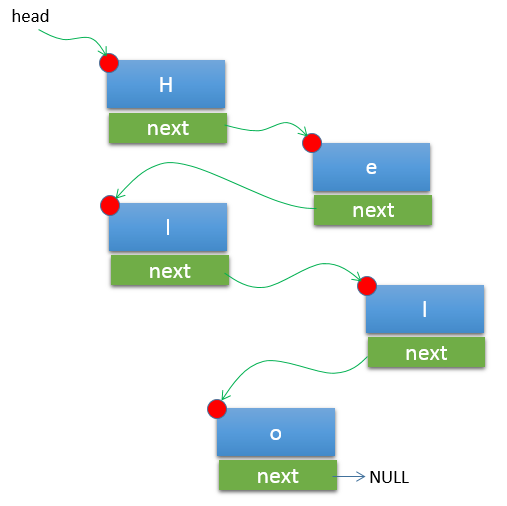Next step is to print out each elements. This would require some efforts to understand, but I would leave it up to you to analyze each step on your own. Give it a try.       node = head;       printf("\n -------Printing the list ------- \n");       while(node != NULL)     {         printf("%c",node->letter);         node = node->next;     };     Example 2 > ----------------------------------------------------------------------------------------------   #include #include   //(1) Define a structure of the node that is used to construct a list struct charList {     char letter;     struct charList *next; };   //(2) Define variables that are used in the process of building up a list struct charList *head = NULL; struct charList *curr = NULL; struct charList *node = NULL;   struct charList* create_list(char letter) {     printf("\ncreating list with headnode as [%c]\n",letter);       node = (struct charList*)malloc(sizeof(struct charList));     if(NULL == node)     {         printf("\n Node creation failed \n");         return NULL;     }     node->letter = letter;     node->next = NULL;       return node; }   struct charList* addItem(char letter) {       node = (struct charList*)malloc(sizeof(struct charList));       node->letter = letter;     node->next = NULL;       curr->next = node;     curr = node;       return node; }   void printList(struct charList *head) {     node = head;       printf("\n -------Printing the list ------- \n");       while(node != NULL)     {         printf("%c",node->letter);         node = node->next;     };       return; };   int main(int argc, char* argv[]) {       head = curr = create_list('H');     addItem('e');     addItem('l');     addItem('l');     addItem('o');       printList(head);       printf("\nPress Any Key to Quit\n");     getchar();          return 0; }     Result > -------Printing the list ------- Hello